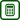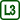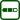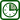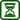## Double-Slit Experiment

### Experiment number : 1703

• #### Goal of experiment

This experiment should help students grasp the term interference and familiarize them with Young's historically important experiment.

• #### Theory

The principle of interference is that two waves coming from different sources to one point superpose in that point. Interference is used for demonstration of the wave nature of the investigated physical phenomenon.

If we want to observe the interference phenomenon, the superposing light waves must be coherent. Light waves are coherent if they have the same frequency and their phase difference is at a considered point of space constant. At the points of interference minima, where waves with opposite phase meet, the light intensity decreases. On the contrary, at the points of interference maxima, where the waves with the same phase meet, the light intensity increases.

If we illuminate a pair of slits with a monochromatic light, an interference pattern with parallel, equidistant dark and light stripes is created on a screen. the distance y between two adjacent maxima (or minima) is directly proportional to the distance l between the screen and the slits and the wavelength λ of the monochromatic light; it is inversely proportional to the distance d between the slits. Thus

$y=\lambda\frac{l}{d}.\tag{1}$
• #### Tools

Laser pointer, three micro pencil graphite refills with a diameter of 0.5 mm, screen.

• #### Procedure

1. We hold the three refills between the thumb and the forefinger of one hand so that they are parallel, side by side, and from a greater part visible.

2. We point the laser pointer at them against the screen.

3. An interference pattern appears on the screen (see photo below).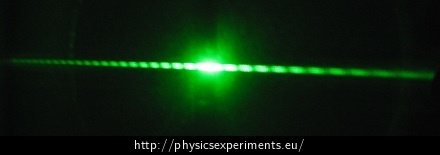• #### Sample result

During this experiment a laser pointer with given wavelength λ = 532 nm was used. The distance between the screen and the refills was set as l =1.5 m. The distance between two interference maxima was measured as y = 1 mm. The distance of the slits can then be calculated from the relation (1).

After substituting into the adjusted equation (1) we obtain an approximate distance of the slits

$d=\frac{l\lambda}{y}= \frac{1.5\,\cdot5.32\,\cdot10^{-7}}{10^{-3}}\,\mathrm{m} \doteq7.98\,\cdot10^{-4}\,\mathrm{m} \doteq0.8\,\mathrm{mm}.$

With respect to the diameter of the refills this result is within the errors, committed in this measurement, considered to be realistic.

• #### Technical Notes

• When working with a laser, it is necessary to follow safety rules.

• A classroom wall can be used as a screen.

• It is convenient to fasten the laser pointer and the refills into holders.

• It is possible to use holders with clamps; one for the laser pointer, the other for the refills tied with a duct tape. The duct tape is used to prevent the refills from breaking and falling apart during the experiment.

• For clearer interference pattern, it is good to darken the class, but this is not essential.

• #### Pedagogical notes

• If we know the distance of the slits, we should be able to determine the wavelength of the laser used or the distance between the slits and the screen using the equation (1).

• Students should realize that the two slits represent two coherent light sources.

• The laser and one refill can also be used to illustrate the bending phenomenon of light on a thin obstacle.